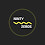# Pandas in Python for Data Analysis with Example(Step-by-Step guide)

### Beginners Pandas Getting Started¶

Pandas is a high-level data manipulation tool developed by Wes McKinney. It is built on the Numpy package and its key data structure is called the DataFrame. DataFrames allow you to store and manipulate tabular data in rows of observations and columns of variables.

pandas is well suited for:
• Tabular data with heterogeneously-typed columns, as in an SQL table or Excel spreadsheet
• Ordered and unordered (not necessarily fixed-frequency) time series data.
• Arbitrary matrix data (homogeneously typed or heterogeneous) with row and column labels
• Any other form of observational / statistical data sets. The data actually need not be labeled at all to be placed into a pandas data structure
Key features:
• Easy handling of missing data
• Size mutability: columns can be inserted and deleted from DataFrame and higher dimensional objects
• Automatic and explicit data alignment: objects can be explicitly aligned to a set of labels, or the data can be aligned automatically
• Powerful, flexible group by functionality to perform split-apply-combine operations on data sets
• Intelligent label-based slicing, fancy indexing, and subsetting of large data sets
• Intuitive merging and joining data sets
• Flexible reshaping and pivoting of data sets
• Hierarchical labeling of axes
• Robust IO tools for loading data from flat files, Excel files, databases, and HDF5
• Time series functionality: date range generation and frequency conversion, moving window statistics, moving window linear regressions, date shifting and lagging, etc.
We’ll start with a quick, non-comprehensive overview of the fundamental data structures in pandas to get you started. The fundamental behavior about data types, indexing, and axis labeling / alignment apply across all of the objects. To get started, import numpy and load pandas into your namespace:
documentation: http://pandas.pydata.org/pandas-docs/stable/10min.html

### Series¶

Series is a one-dimensional labeled array capable of holding any data type (integers, strings, floating point numbers,
Python objects, etc.). The axis labels are collectively referred to as the index.
documentation: http://pandas.pydata.org/pandas-docs/stable/generated/pandas.Series.html

In :
```#importing numpy and pandas library
import pandas as pd
import numpy as np
```

#### Create series from NumPy array¶

Creating a basic series from NumpPy array.
Number of labels in 'index' must be the same as the number of elements in array

In :
```my_simple_series = pd.Series(np.random.randn(7), index=['a', 'b', 'c', 'd', 'e','f','g'])
my_simple_series
```

Out:
```a    0.623720
b    0.397227
c    0.470759
d    0.323920
e   -1.186631
f   -1.175695
g    0.744503
dtype: float64```

In :
```my_simple_series.index
```

Out:

`Index([u'a', u'b', u'c', u'd', u'e', u'f', u'g'], dtype='object')`

#### Create series from NumPy array, without explicit index¶

In :
```my_simple_series = pd.Series(np.random.randn(5))
my_simple_series
```

Out:

```0    1.285379
1   -0.672387
2   -0.720461
3   -0.263968
4    0.547311
dtype: float64```

Access a series like a NumPy array

In :
```my_simple_series[:3]
```

Out:
```0    1.285379
1   -0.672387
2   -0.720461
dtype: float64```

##### Create series from Python dictionary¶
In :
```my_dictionary = {'a' : 45., 'b' : -19.5, 'c' : 4444}
my_second_series = pd.Series(my_dictionary)
my_second_series
```

Out:
```a      45.0
b     -19.5
c    4444.0
dtype: float64```

Access a series like a dictionary

In :
```my_second_series['b']
```

Out:

`-19.5`

note order in display; same as order in "index"
note NaN

In :
```pd.Series(my_dictionary, index=['b', 'c', 'd', 'a'])
```

Out:

```b     -19.5
c    4444.0
d       NaN
a      45.0
dtype: float64```

In :
```my_second_series.get('a')
```

Out:

`45.0`

In :
```unknown = my_second_series.get('f')
type(unknown)
```

Out:

`NoneType`

##### Create series from scalar¶
If data is a scalar value, an index must be provided. The value will be repeated to match the length of index

In :
```pd.Series(5., index=['a', 'b', 'c', 'd', 'e'])
```

Out:

```a    5.0
b    5.0
c    5.0
d    5.0
e    5.0
dtype: float64```

### Vectorized Operations¶

• not necessary to write loops for element-by-element operations
• pandas' Series objects can be passed to MOST NumPy functions
documentation: http://pandas.pydata.org/pandas-docs/stable/basics.html

In :
```my_dictionary = {'a' : 45., 'b' : -19.5, 'c' : 4444}
my_series = pd.Series(my_dictionary)
my_series
```

Out:

```a      45.0
b     -19.5
c    4444.0
dtype: float64```

In :
```my_series + my_series
```

Out:

```a      90.0
b     -39.0
c    8888.0
dtype: float64```

In :
```my_series
```

Out:

```a      45.0
b     -19.5
c    4444.0
dtype: float64```

##### Series within arithmetic expression¶
In :
```#adding values into a series
my_series +5
```

Out:

```a      50.0
b     -14.5
c    4449.0
dtype: float64```

##### Series used as argument to NumPy function¶
In :
```np.exp(my_series)
```

Out:

```a    3.493427e+19
b    3.398268e-09
c             inf
dtype: float64```

A key difference between Series and ndarray is that operations between Series automatically align the data based on
label. Thus, you can write computations without giving consideration to whether the Series involved have the same labels.

In :
```my_series[1:]
```

Out:

```b     -19.5
c    4444.0
dtype: float64```

In :
```my_series[:-1]
```

Out:

```a    45.0
b   -19.5
dtype: float64```

In :
```my_series[1:] + my_series[:-1]
```

Out:

```a     NaN
b   -39.0
c     NaN
dtype: float64```

### Apply Python functions on an element-by-element basis¶

In :
```def multiply_by_ten (input_element):
return input_element * 10.0
```

In :
```my_series.map(multiply_by_ten)
```

Out:

```a      450.0
b     -195.0
c    44440.0
dtype: float64```

### Vectorized string methods¶

Series is equipped with a set of string processing methods that make it easy to operate on each element of the array. Perhaps most importantly, these methods exclude missing/NA values automatically.

In :
```series_of_strings = pd.Series(['A', 'B', 'C', 'Aaba', 'Baca', np.nan, 'CABA', 'dog', 'cat'])
```

In :
```series_of_strings.str.lower()
```

Out:

```0       a
1       b
2       c
3    aaba
4    baca
5     NaN
6    caba
7     dog
8     cat
dtype: object```

#### In the next post we will continue seeing the arithmetic Operations, So Subscribe it and Stay tuned!¶

Please Subscribe and Share with fellow developer!

• Reference resource :

#### Venkat

Hey I'm Venkat
Developer, Blogger, Thinker and Data scientist. nintyzeros [at] gmail.com I love the Data and Problem - An Indian Lives in US .If you have any question do reach me out via below social mediaBalas

Wow...Nyc post to get started with series!Balas